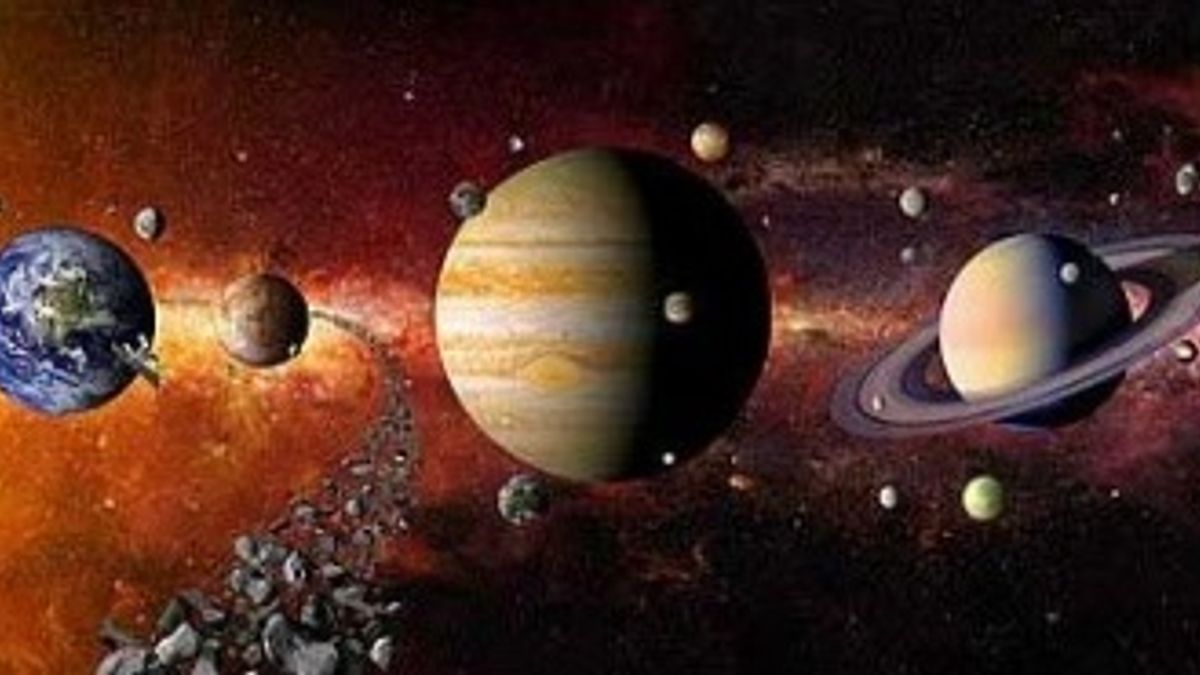# How Gravitational Force holds the Solar System together?

The gravitational force of earth is responsible for holding the atmosphere above the earth. It is also the gravitational force of earth which keeps us firmly on the ground. Various objects attract each other due to gravity only. This article deals what is gravitational force and how this force holds the Solar System together.How Gravitational Force holds the Solar System together

As we know that force is necessary to produce motion in a body. If any object falls from some height towards the earth, therefore a force must be acting on it. This force is due to the attraction between the earth and the object which is known as Gravitational Force of earth or gravity of earth. Similarly, a leaf falls down from a tree due to gravity of earth. In fact earth attracts or pulls all the objects towards its centre.
The gravitational force of the earth is responsible for holding the atmosphere above the earth; for the rain falling to the earth; and for the flow of water in the rivers. It is also the gravitational force of earth, which keeps us firmly on the ground. Though the various objects on this earth attract one another constantly, they do not cause any motion because the gravitational force of attraction between them is very small. When two bodies or objects are very big, having large masses, then the gravitational force of attraction between them becomes extremely large. For example the sun, the moon and the earth have extremely large masses, therefore, the gravitational force of attraction between the sun and the earth or other planets or between the earth and the moon, is extremely large. Through this article we will discuss how gravitational force holds the solar system together.
First it is necessary to understand that every object in the Universe attracts every other object

Source: www.image.slidesharecdn.com

Do you know why Saturn have rings and how they are formed?
We know that when we drop a ball from a certain height, it falls towards the earth. This means that the earth attracts the various objects towards its centre. According to Newton, it is not only the earth, which attracts the objects, in fact, every object attracts every other object with a certain force. The force with which two objects attract each other is called gravitational force or gravity. The gravitational force between two objects, acts even if the two objects are not connected by any means.
But if the masses of the objects are small, then the gravitational force between them is very small which cannot be detected easily. For example, two stones lying on the ground attract each other, but since their masses are small, the gravitational force of attraction between them is small and hence we do not see one stone moving towards the other stone. If, however, one of the objects is very big having a large mass, then the gravitational force becomes very large and its effect can be seen easily.
How gravitational force holds the solar system together?
In the Solar System, planets move in circular orbits around the sun; and satellites move in circular orbits around the planets. A centripetal force is needed to make an object move in a circular orbit or circular path. Now,the question arises what is centripetal force?

Source: www.wikimedia.org.com
A centripetal force is not a fundamental force, but a net force which causes an object to move in a circular path. It is directed towards the centre around which the body is moving. In the case of planets moving around the sun, the centripetal force is provided by the gravitational force of the sun. And in the case of satellites moving around the planets, the centripetal force is provided by the gravitational force of the planets.
In the case of ‘the sun and the earth’
Since the masses of the sun and the earth are very, very large, they exert very large force on one another. It is the gravitational force between the sun and the earth, which keeps the earth in uniform circular motion around the sun.
In the case of ‘the earth and the moon’
It is the gravitational force between the earth and the moon that makes the moon revolve at uniform speed around the earth.
Thus, the gravitational force is responsible for the existence of our solar system. Due to this force motion of planets around the sun; motion of the moon around the earth; formation of tides in the sea etc. took place or can be explained.
From the above article it is clear that what is gravitational force, centripetal force, how gravitational force holds the solar system together.

Do you know that our moon is made up of 20 smaller moonlets?

Get the latest General Knowledge and Current Affairs from all over India and world for all competitive exams.
खेलें हर किस्म के रोमांच से भरपूर गेम्स सिर्फ़ जागरण प्ले पर